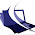## Sunday, 18 August 2013

### First and Last Method in LINQ

Written By:-Isha Malhotra
Our Website:-www.techaltum.com

First and Last in LINQ

Use of First in LINQ

First method in LINQ just select the top 1 value from the data source.

For Example:-

First with array

int[] marks = new int[] { 23, 45, 78, 45, 56, 89, 56, 32 };

int first = marks.First();

Response.Write("First Element " + first);
Ouput

We can also pass condition while calling first method

For example:-

int[] marks = new int[] { 23, 45, 78, 45, 56, 89, 56, 32 };

int first = marks.First(x=>x%2==0);

Response.Write("First Element " + first);

It will simply show 78.

Similarly we can implement the same code on class type list.

For example:-

Note:-data is class which contains variable roll_no, student and per.

List<data> dt = new List<data>()
{
new data{roll_no=1, student="isha", per=100},
new data{roll_no=2, student="rahul", per=34},
new data{roll_no=2, student="rahul", per=34},
new data{roll_no=5, student="renu", per=34},
new data{roll_no=5, student="sapna", per=89}
};

data res = dt.First();

Response.Write("Roll No:- " + res.roll_no + " Student:- " + res.student + "  per:- " + res.per + "<br/>");

And if you want to put any condition then you can add your condition which is as follows:-

data res = dt.First(x=>x.per>90);

Use of Last in LINQ

Last method in LINQ just select the last value from the data source.

For example:-

int[] marks = new int[] { 23, 45, 78, 45, 56, 89, 56, 32 };

int Last = marks.Last();

Response.Write("Last Element " + Last);

As you try example in first same example you can try with last. The difference is that it will show last element.

#### 1 comment:

1.Really something Grate in this article Thanks for sharing this. We are providing Online Training Classes. After reading this slightly I am changed my way of introduction about my training to people.

Best Linux training in Noida
Linux Training Institute in Noida